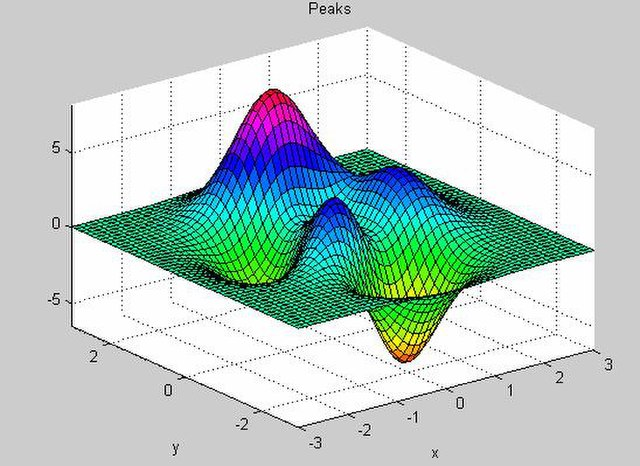# Numerical analysisI am interested in designing numerical schemes (mostly finite difference schemes) that preserve the physical properties of the underlying physical models.

• Schemes for dispersive equations.
• Finite differences for Maxwell-Debye and Maxwell-Lorentz equations.
• Finite differences for Maxwell-Bloch equations.
• Finite volumes for Maxwell equations.
• Splitting and nonstandard methods.

Part of my Habilitation thesis deals with the theme. I also wrote a master-level book on models in nonlinear and quantum optics, including numerical issues.

Ongoing works deal with asymptotic preserving schemes for Bloch equations.